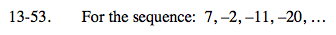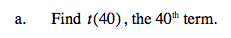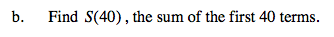### Home > A2C > Chapter Ch13 > Lesson 13.1.3 > Problem13-53

13-53.
1. For the sequence: 7, −2, −11, −20, … Homework Help ✎

1. Find t(40), the 40th term.

2. Find S(40), the sum of the first 40 terms.t(n) = −9n + 16

t(40) = −344Use the summation formula:

$S_{40}=\frac{40(t(1)+t(40))}{2}$